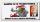Whole numbers

Pavol wrote down a number that is both rational and a whole number. What is one possible number she could have written down?

Result

x =  5

Solution:Leave us a comment of this math problem and its solution (i.e. if it is still somewhat unclear...):Be the first to comment!Next similar math problems:

1. Double 5Peter was thinking of a number. Peter doubles it and gets an answer of 8.6. What was the original number?
2. A numberA number increased by 7.9 is 8.3
3. Reduce to lowest termsReduce to lowest terms : 32/124
4. Equation 11Solve equation: ?
5. PresidentPresident of Slovakia earns a monthly € 7844 per month. How many times earn than Jimmy's monther salary € 612? 15.3.2014 the presidential election, which decides who will almost effortlessly 5 years to receive such space salary for nothing;)
6. Two numbers 6Fill two natural numbers a, b: 7 + blank- blank = 5
7. Write decimalsWrite in the decimal system the short and advanced form of these numbers: a) four thousand seventy-nine b) five hundred and one thousand six hundred and ten c) nine million twenty-six
8. Mixed2improperWrite the mixed number as an improper fraction. 166 2/3
9. Rounding 2Round the following negative number: -143.021
10. Forest nurseryIn the forest nursery after winter, they found that 1/10 stems died out of them. For them, they land 193 new spruces. How many spruces are in the forest nursery?
11. EquatiomSolve equation with negatives: X/(-5) + 2 = -9
12. Simple equation 8Solve the following equation: 36=-(1+7x)-6(-7-x)
13. ExpressionSolve for a specified variable: P=a+4b+3c, for a
14. In fractionsAn ant climbs 2/5 of the pole on the first hour and climbs 1/4 of the pole on the next hour. What part of the pole does the ant climb in two hours?
15. ZdeněkZdeněk picked up 15 l of water from a 100-liter full-water barrel. Write a fraction of what part of Zdeněk's water he picked.
16. Lengths of the poolMiguel swam 6 lengths of the pool. Mat swam 3 times as far as Miguel. Lionel swam 1/3 as far as Miguel. How many lengths did mat swim?
17. Fraction and a decimalWrite as a fraction and a decimal. One and two plus three and five hundredths